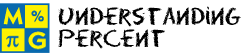# Understanding PercentThis page lists the Learning Objectives for all lessons in Unit 4.

#### Meaning of Percent

The student will be able to:

• Define ratio and percent.
• Describe the relationship between ratios, fractions, decimals and percents.
• Identify the decimal equivalent of a percent.
• Identify the fractional equivalent of a percent.
• Label percentages with the symbol %.
• Apply percent concepts to complete five interactive exercises.

#### Writing Fractions as Percents

The student will be able to:

• Define numerator, denominator and equivalent fraction.
• Convert a fraction to a percent using equivalent fractions.
• Convert a fraction to a percent using division.
• Describe the methods for converting a fraction to a percent.
• Predict the next fraction given a sequence of percents and their fractional equivalents.
• Apply fraction-to-percent conversion procedures to complete five interactive exercises.

#### Writing Decimals as Percents

The student will be able to:

• Convert decimals with varying place values to percents.
• Describe the method for converting a decimal to a percent.
• Explain the connection between place value and decimal-to-percent conversions.
• Apply decimal-to-percent conversion procedures to complete five interactive exercises.

#### Writing Percents as Decimals

The student will be able to:

• Convert whole-number and decimal percents to decimal numbers.
• Describe the procedure for converting a percent to a decimal.
• Explain the connection between place value and percent-to-decimal conversions.
• Connect percents and decimals with money.
• Apply percent-to-decimal conversion procedures to complete five interactive exercises.

#### Writing Percents as Fractions

The student will be able to:

• Define greatest common factor.
• Convert whole-number and decimal percents to fractions.
• Describe the greatest common factor method for reducing fractions to lowest terms.
• Describe the procedure for writing a percent as a fraction in lowest terms.
• Apply percent-to-fraction conversion procedures to complete five interactive exercises.

#### Percents Less Than 1 or Greater than 100

The student will be able to:

• Examine percents less than 1 and percents greater than 100.
• Examine examples in which percents less than 1 are converted to decimal numbers.
• Examine examples in which percents greater than 100 are converted to decimal numbers.
• Examine examples in which percents less than 1 are converted to fractions in lowest terms.
• Examine examples in which percents greater than 100 are converted to fractions in lowest terms.
• Convert a percent less than 1 to a decimal, and to a fraction in lowest terms.
• Convert a percent greater than 100 to a decimal, and to a fraction in lowest terms.
• Recognize that a percent less than one may include a leading zero.
• Recognize that the leading zero reminds us that this number is between 0 and 1 percent.
• Apply conversion procedures to complete five interactive exercises.

#### Practice Exercises

The student will be able to:

• Examine ten interactive exercises for all topics in this unit.
• Determine which concepts and procedures are needed to complete each practice exercise.
• Compute answers by applying appropriate formulas and procedures.
• Self-assess knowledge and skills acquired from this unit.

#### Challenge Exercises

The student will be able to:

• Evaluate ten interactive exercises with word problems for all topics in this unit.
• Analyze each problem to identify the given information.
• Identify the concepts and procedures needed to find the missing value.
• Apply percent conversion concepts to complete each exercise.
• Synthesize all information presented in this unit.

#### Solutions

The student will be able to:

• Examine the solution for each exercise presented in this unit.
• Identify which solutions need to be reviewed.
• Compare solutions to completed exercises.
• Identify and evaluate incorrect answers.
• Amend and label original answers.
• Identify areas of strength and weakness.
• Decide which concepts, formulas and procedures need to be reviewed from this unit.

### Order your CD today for Home or SchoolOR